# NCERT Exemplar Solutions for Class 10 Maths Chapter 9 - Circles

## NCERT Exemplar Solutions Class 10 Maths Chapter 9 – Free PDF Download

NCERT Exemplar Class 10 Maths Chapter 9 Circles, provided here for students to download the PDF format of it and prepare for CBSE exams. These exemplars problems and solutions are designed by our experts in accordance with the latest CBSE Syllabus (2021-2022). Using these solved questions in exemplars, students will be able to clear all their doubts regarding the topics and they will also find detailed answers to all the chapter questions which they can refer and study effectively for the exams. They can also refer to these materials to prepare for competitive exams in the future. Click here to get exemplars for all chapters.

In Chapter 9 students will explore the topic of “circle” and learn about topics like tangent and its existence to a circle. Students will also come across several examples and activities in the chapter which they have to understand and solve. Thus, to facilitate easy learning and help students grasp all the concepts of circles properly, free NCERT exemplar for Chapter 9 of Class 10 Maths is available here. This chapter covers the following topics of circles given below:

• Tangent to a given circle
• Number of tangents drawn from a point on a circle
• Proof of -Tangents at the end of the diameter of a circle are parallel

Class 10 Maths Chapter 9 NCERT Exemplar PDF can be viewed below.

## Download PDF of NCERT Exemplar Solutions for Class 10 Maths Chapter 9 Circles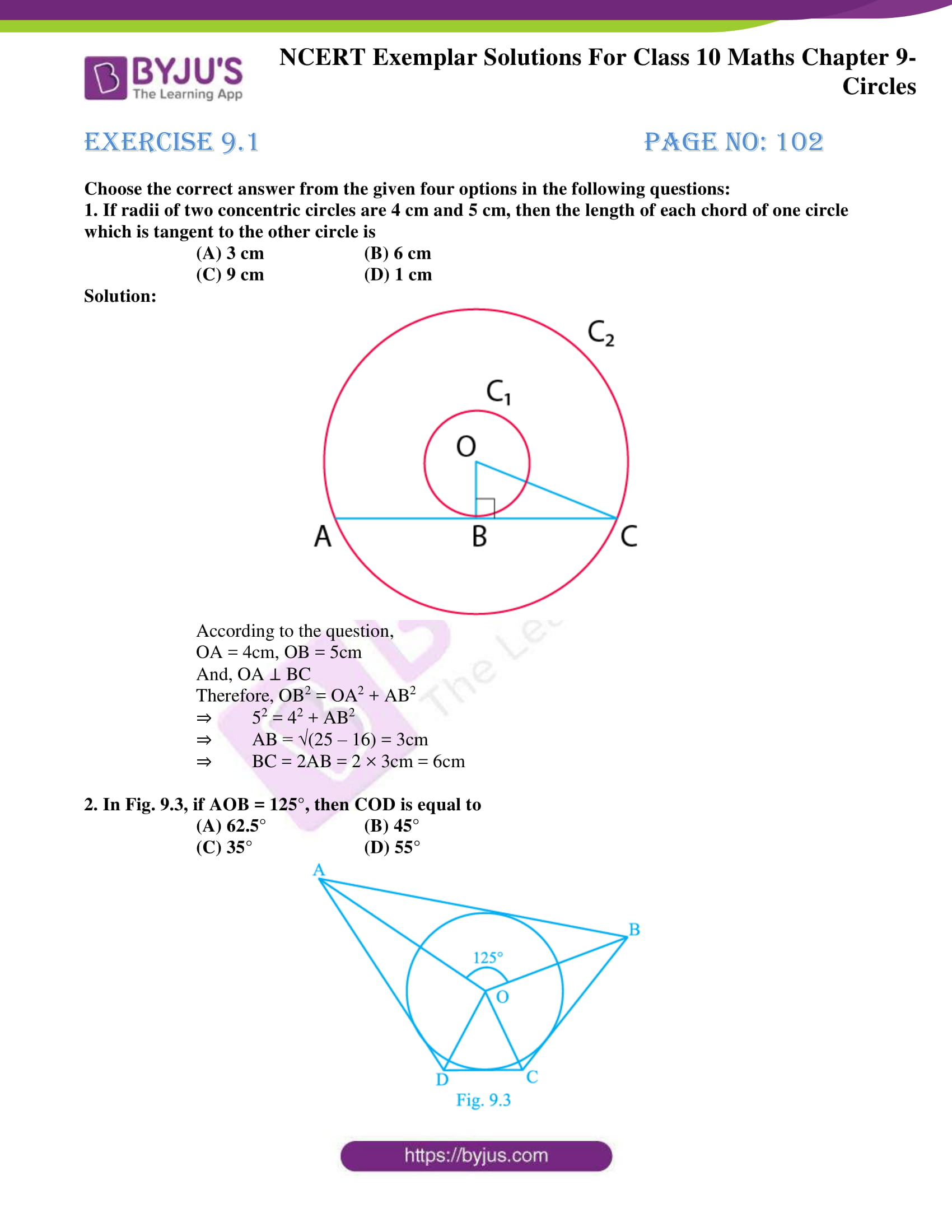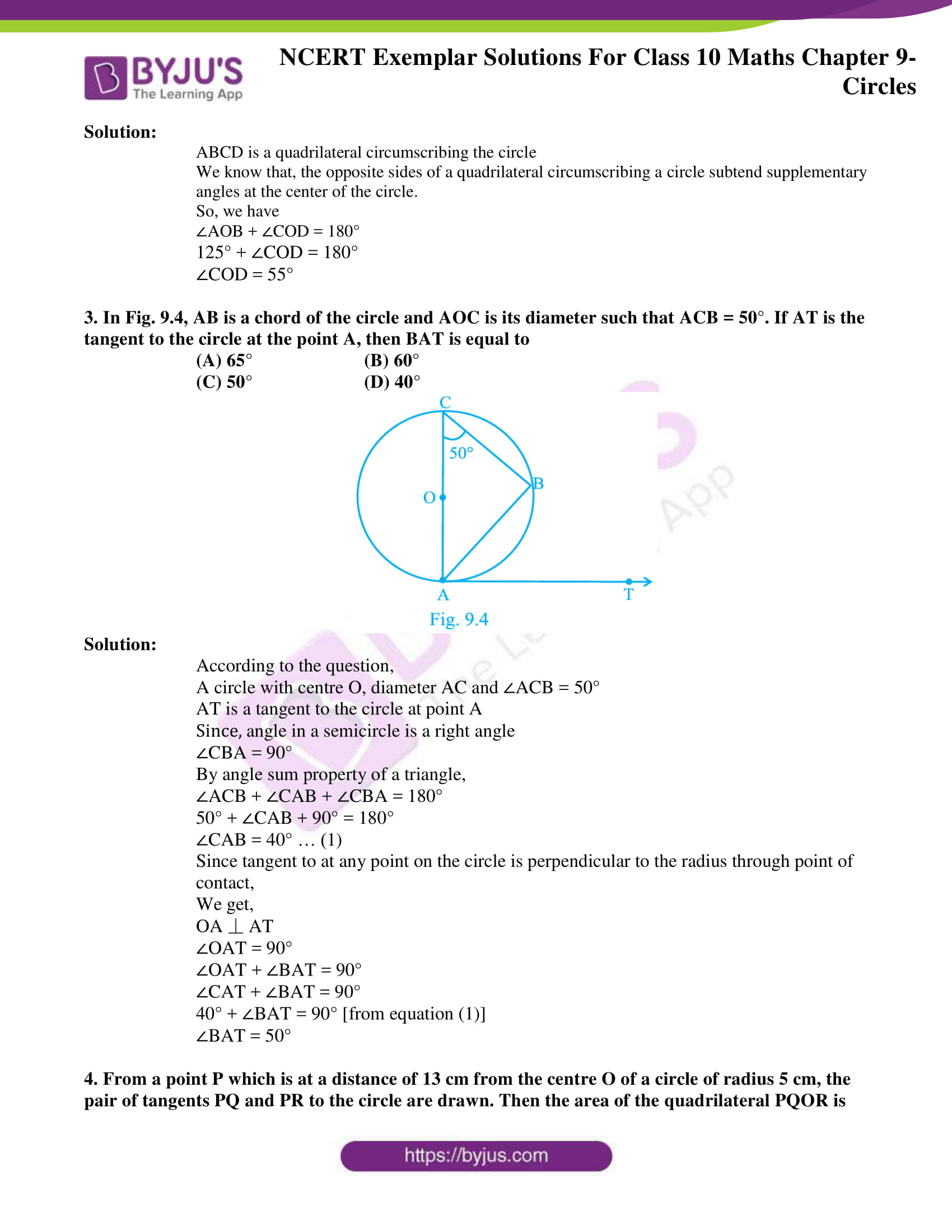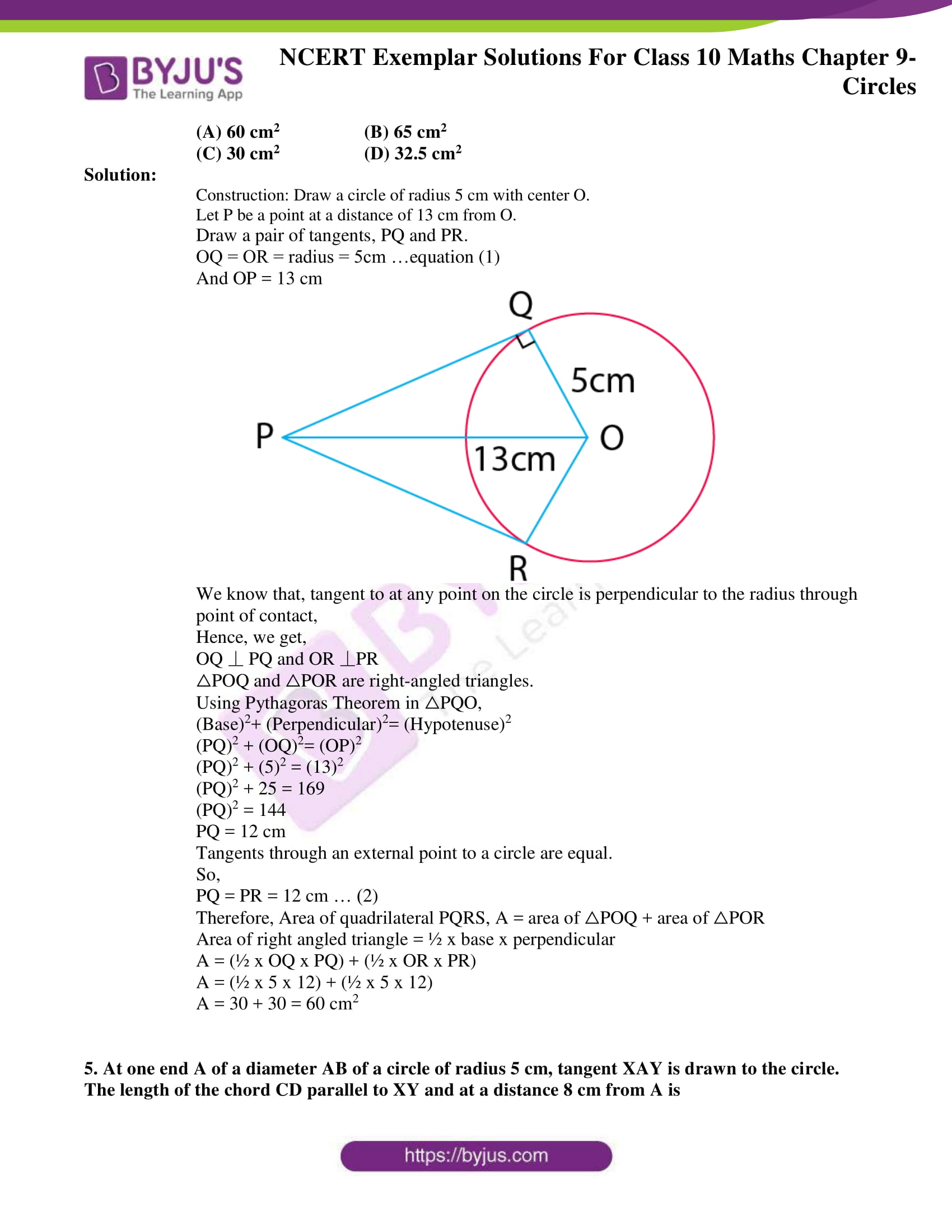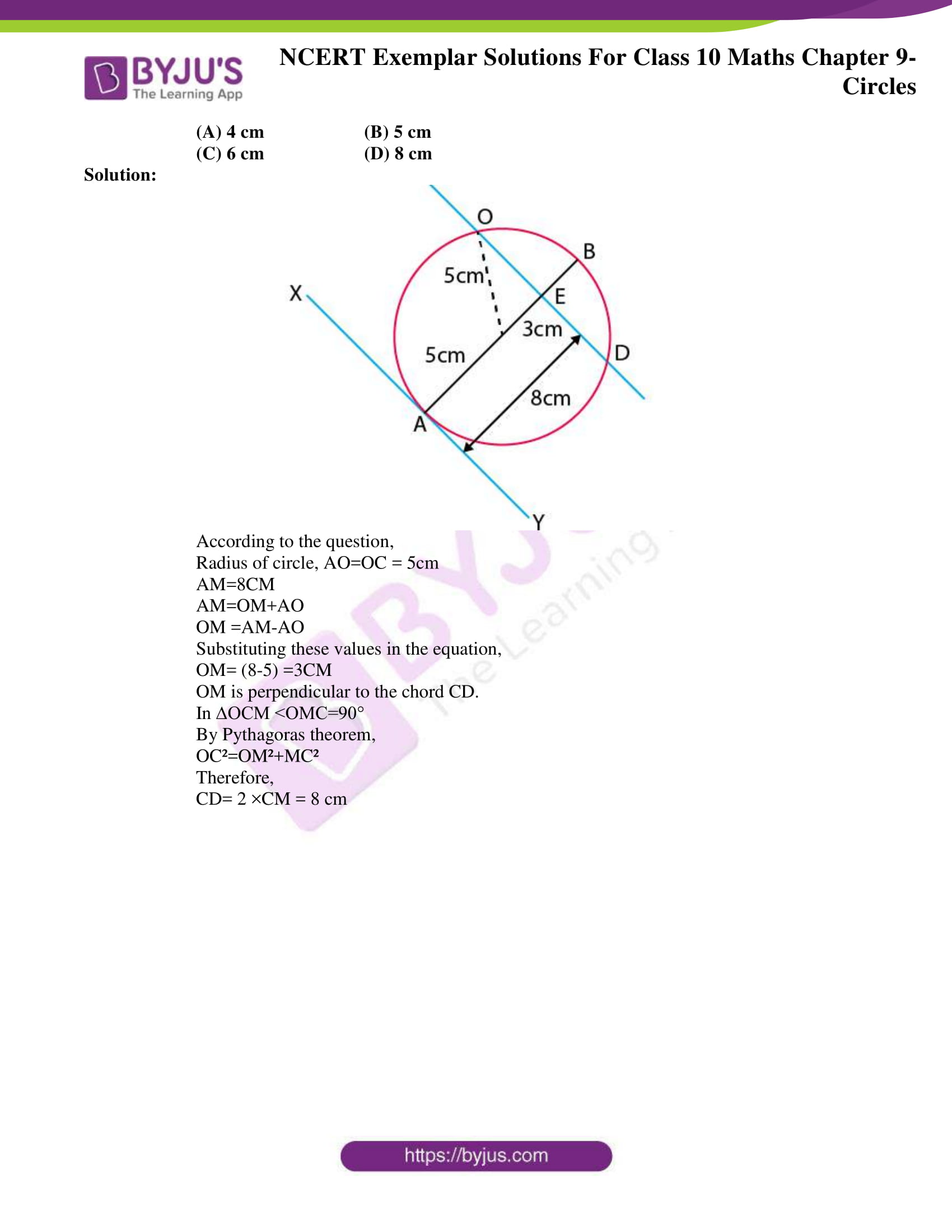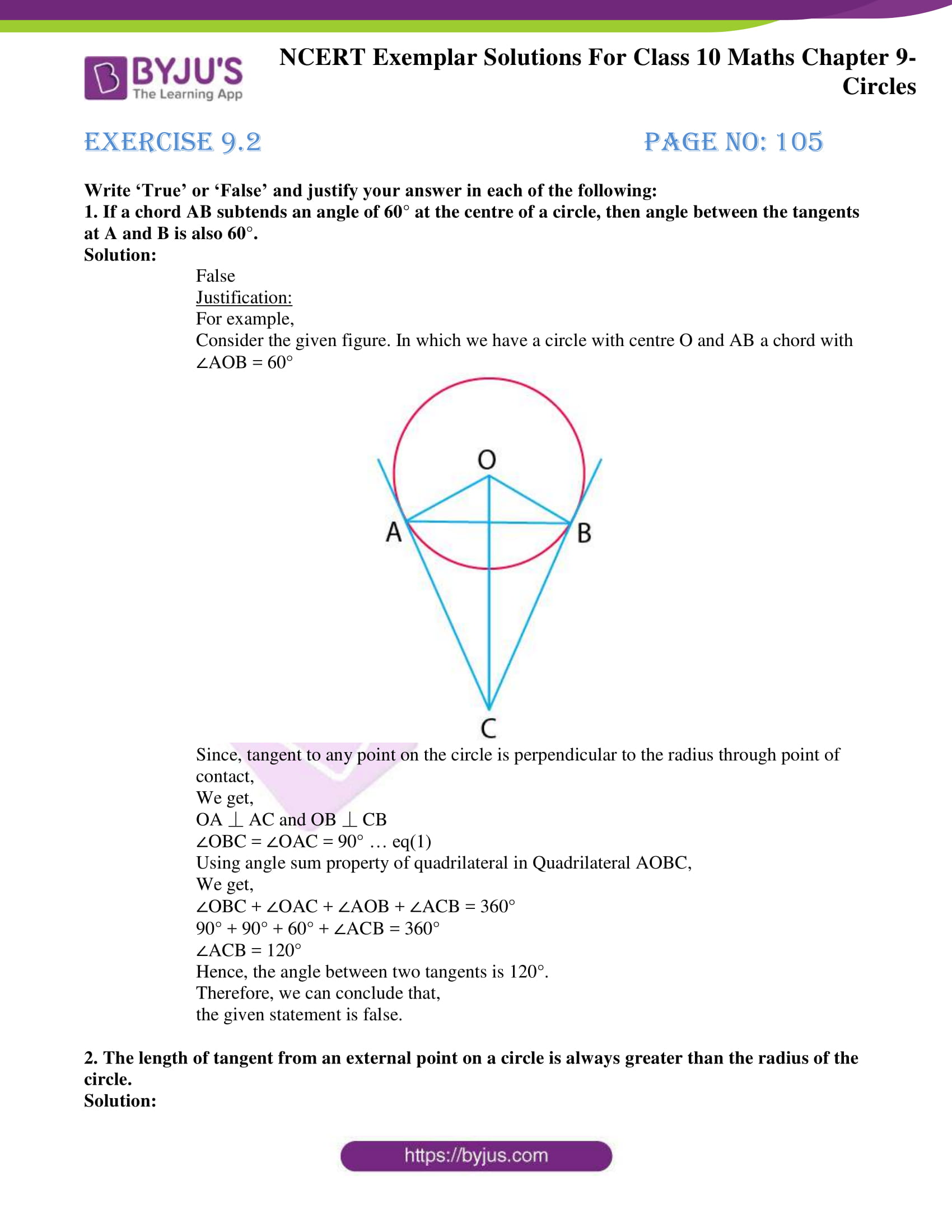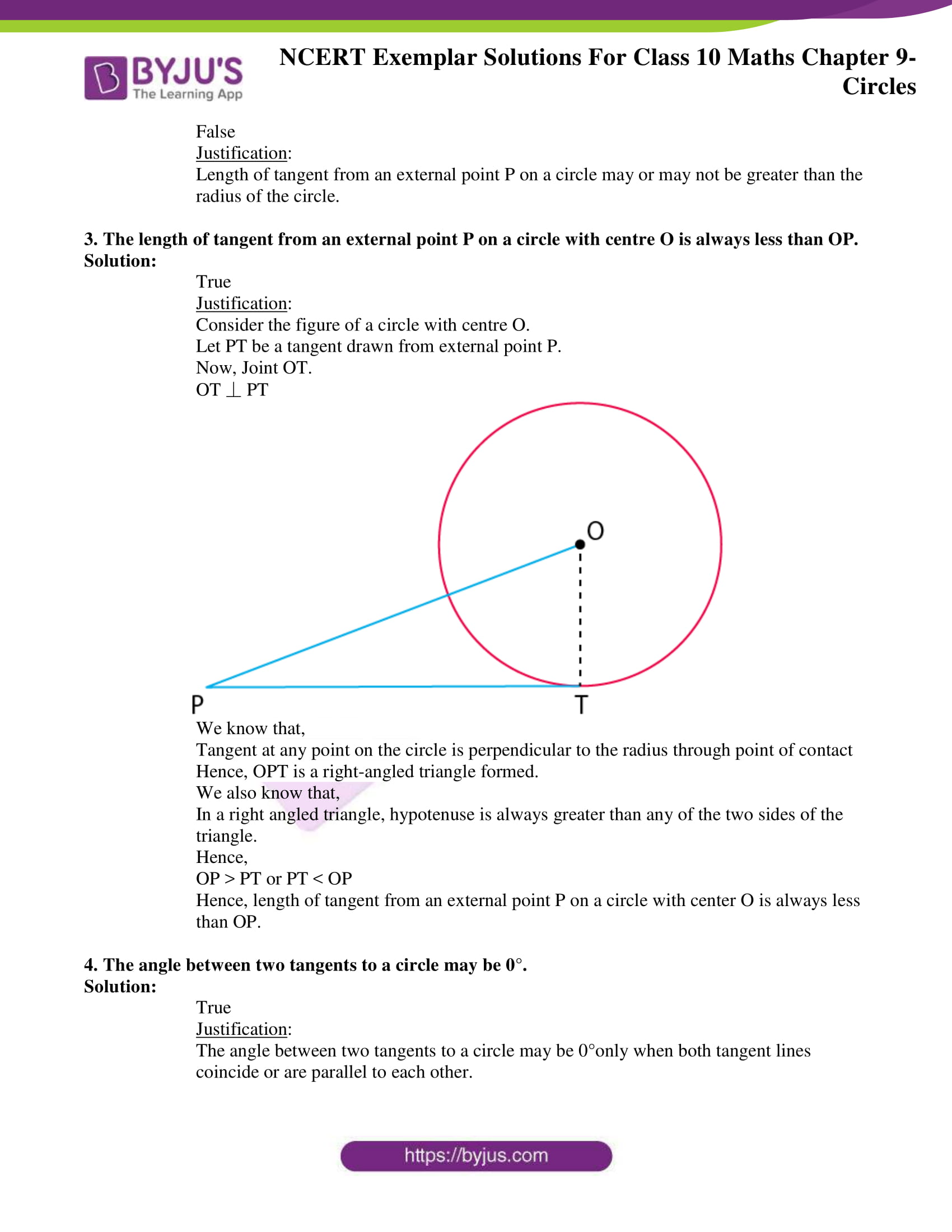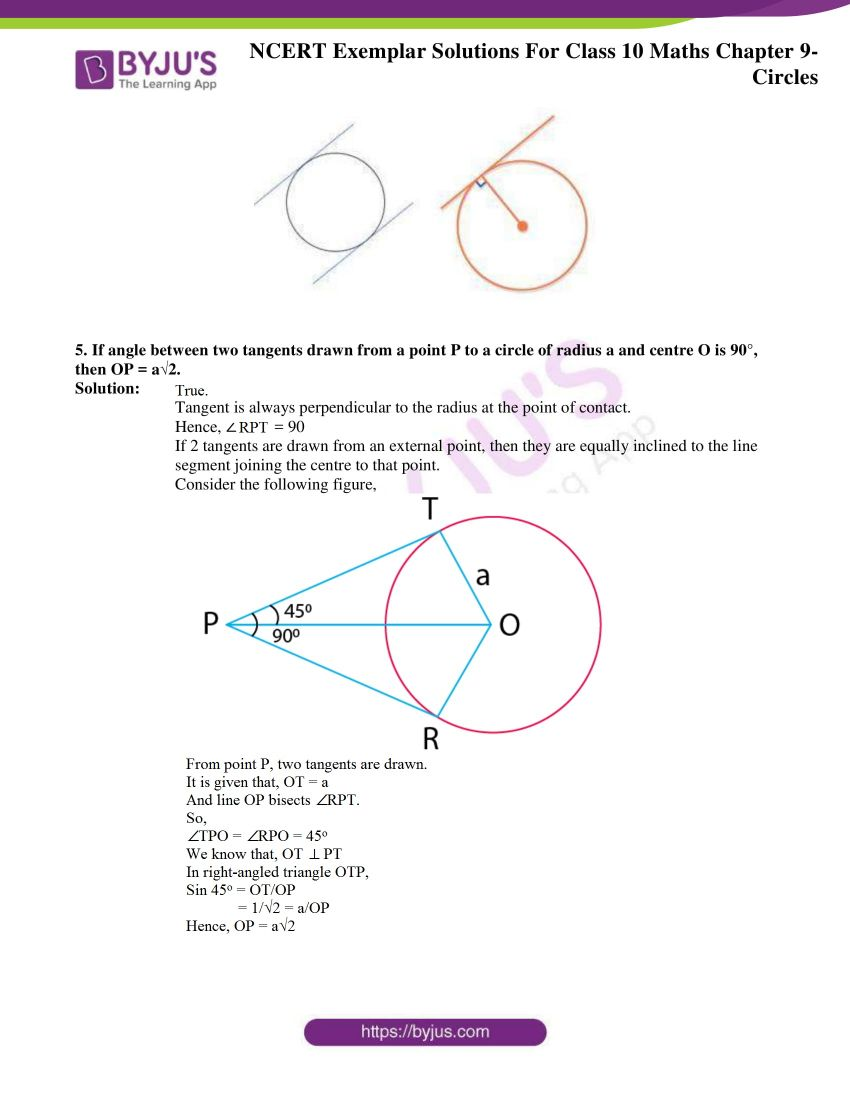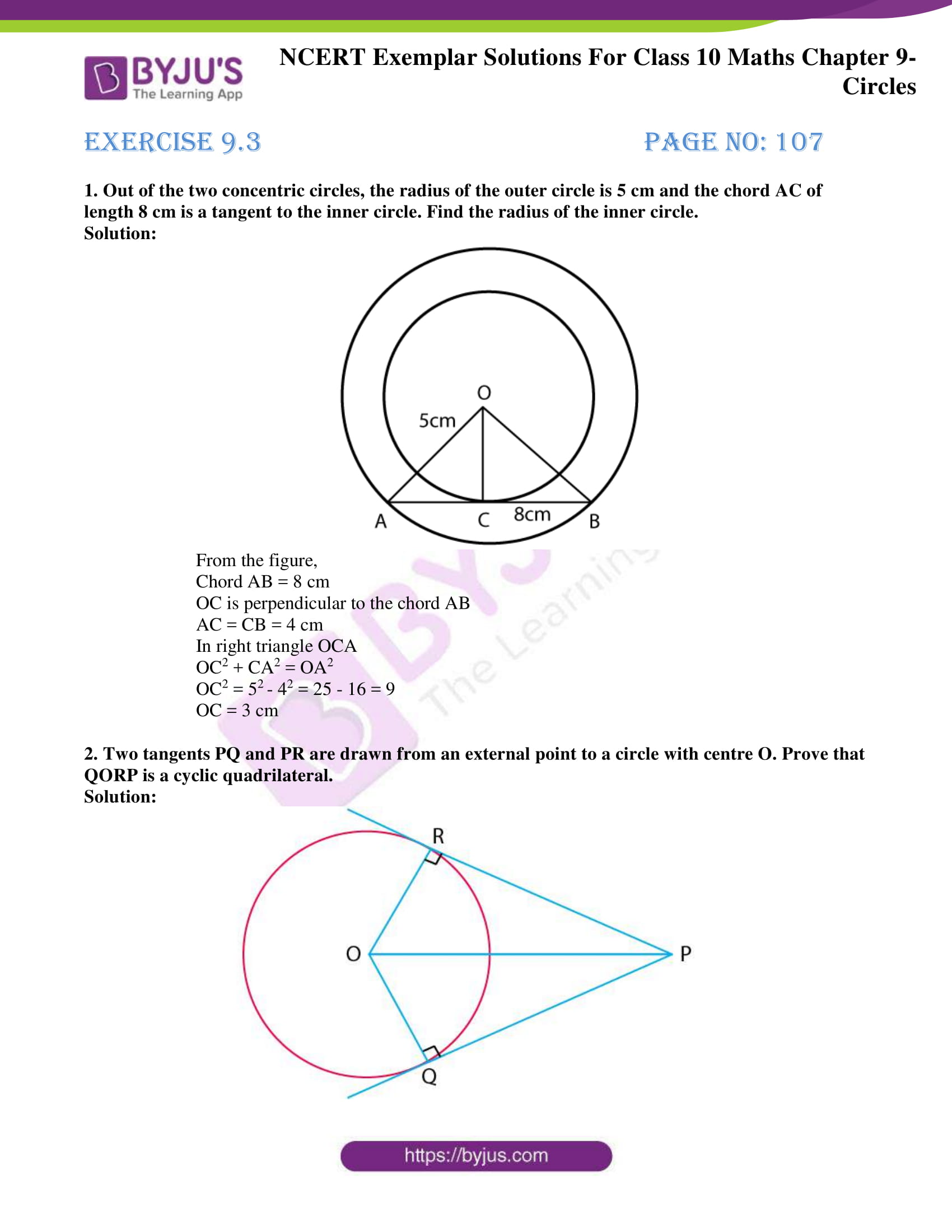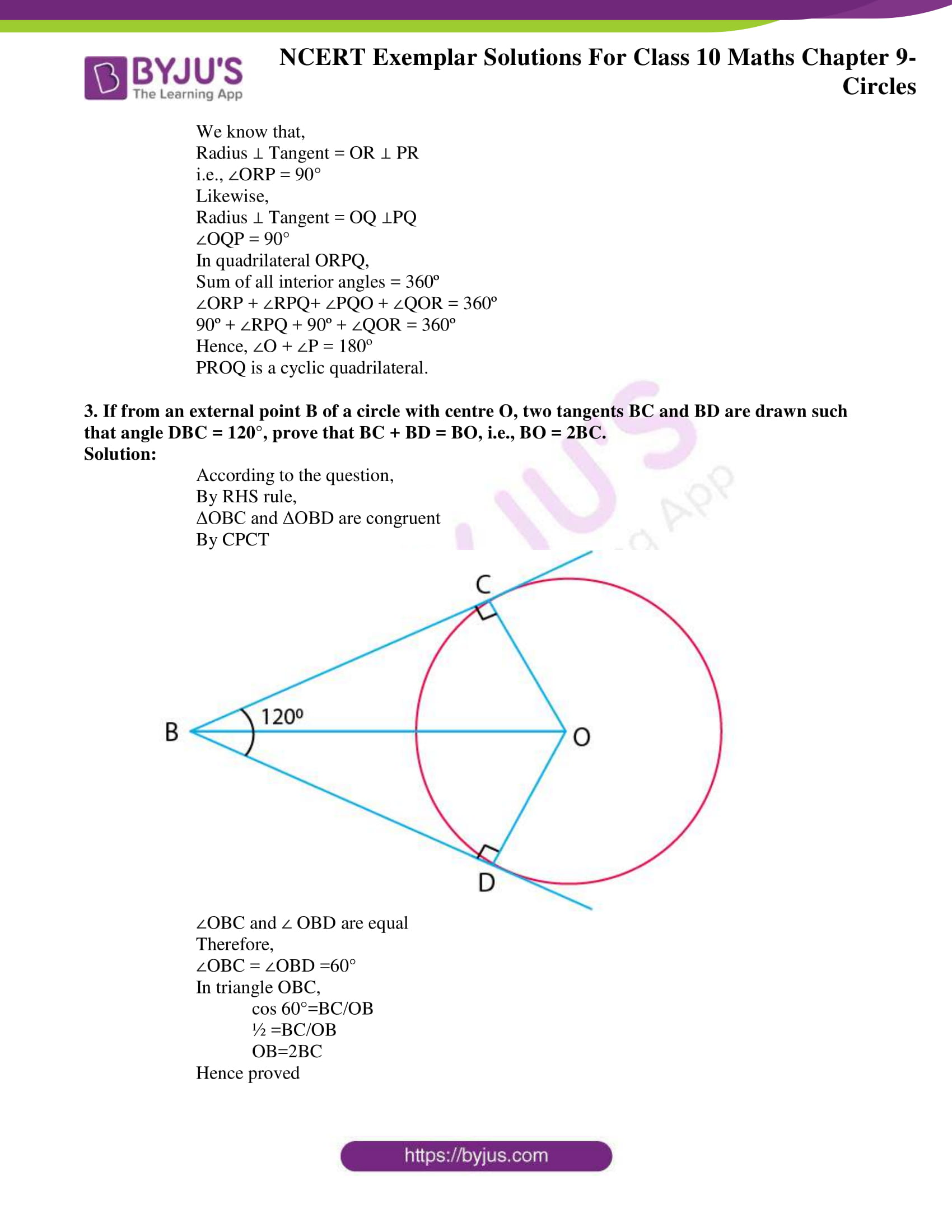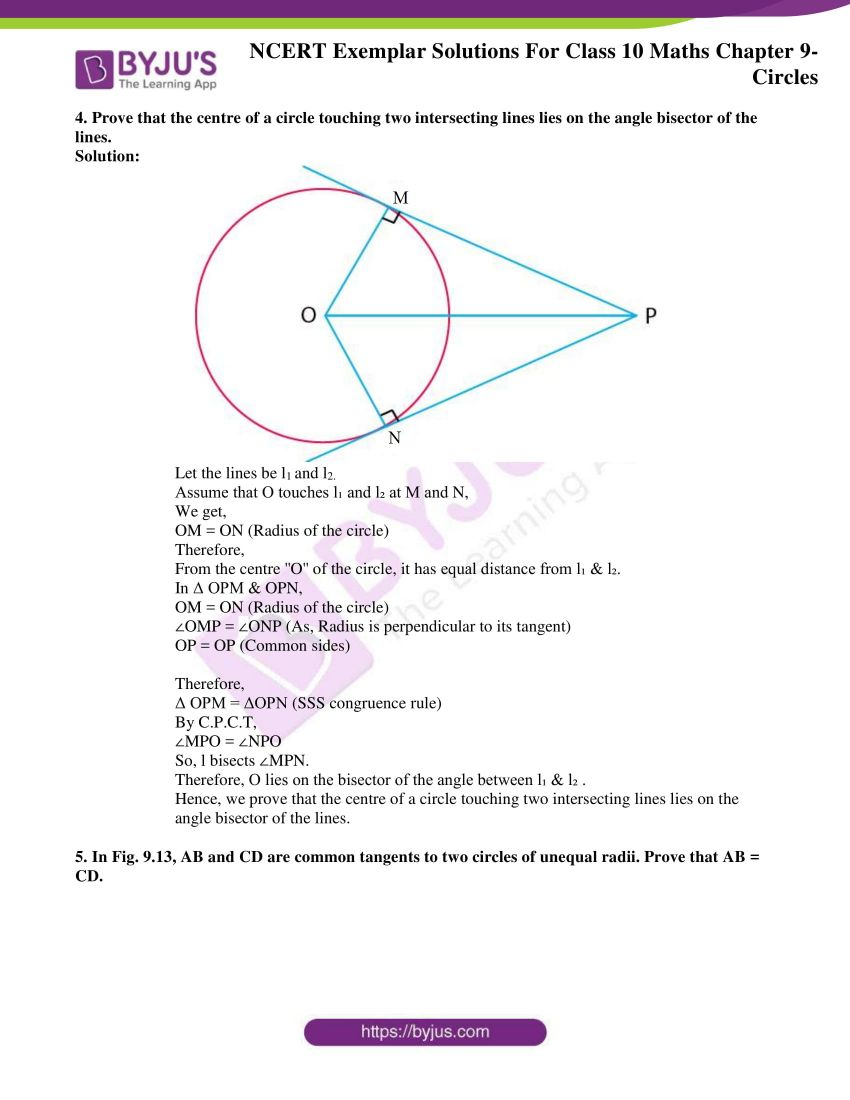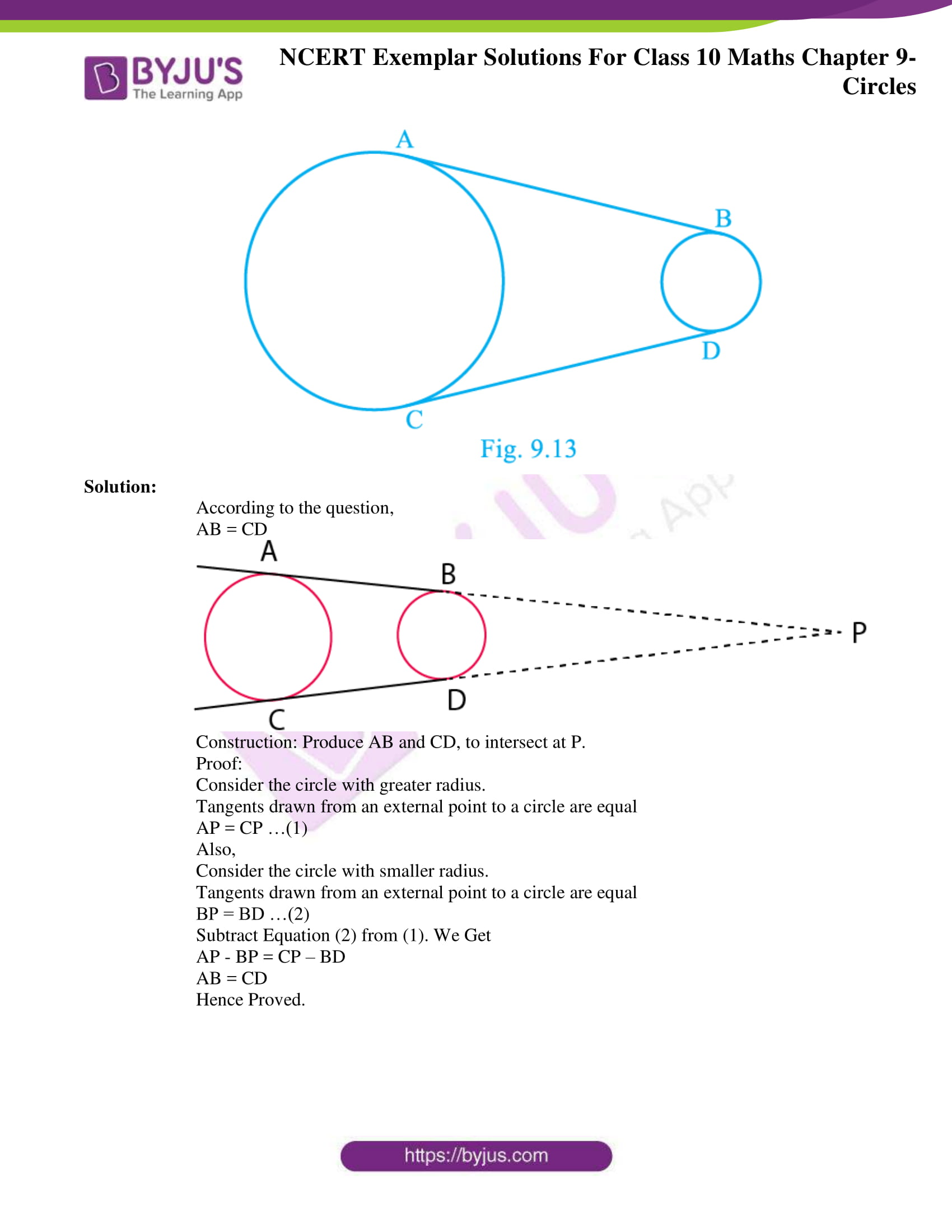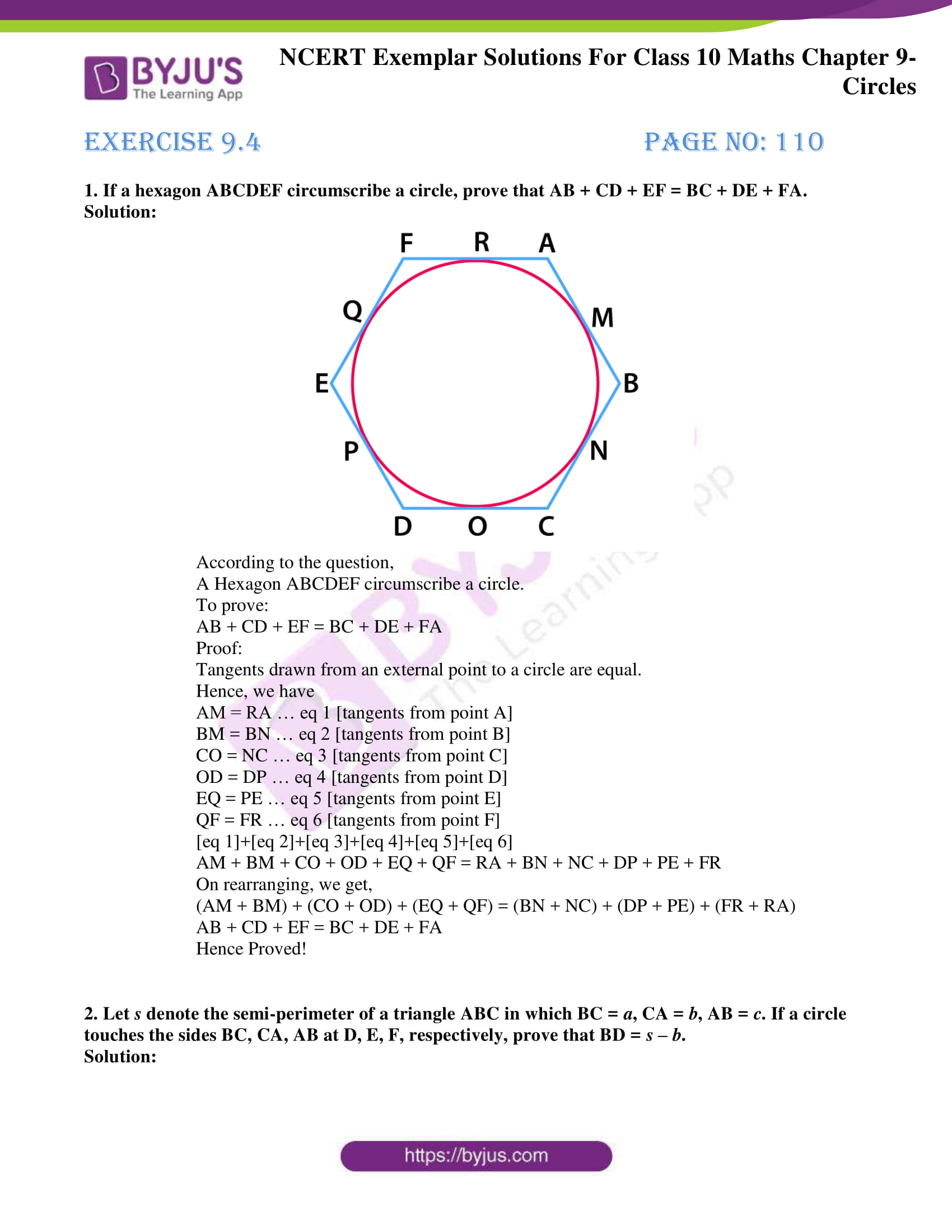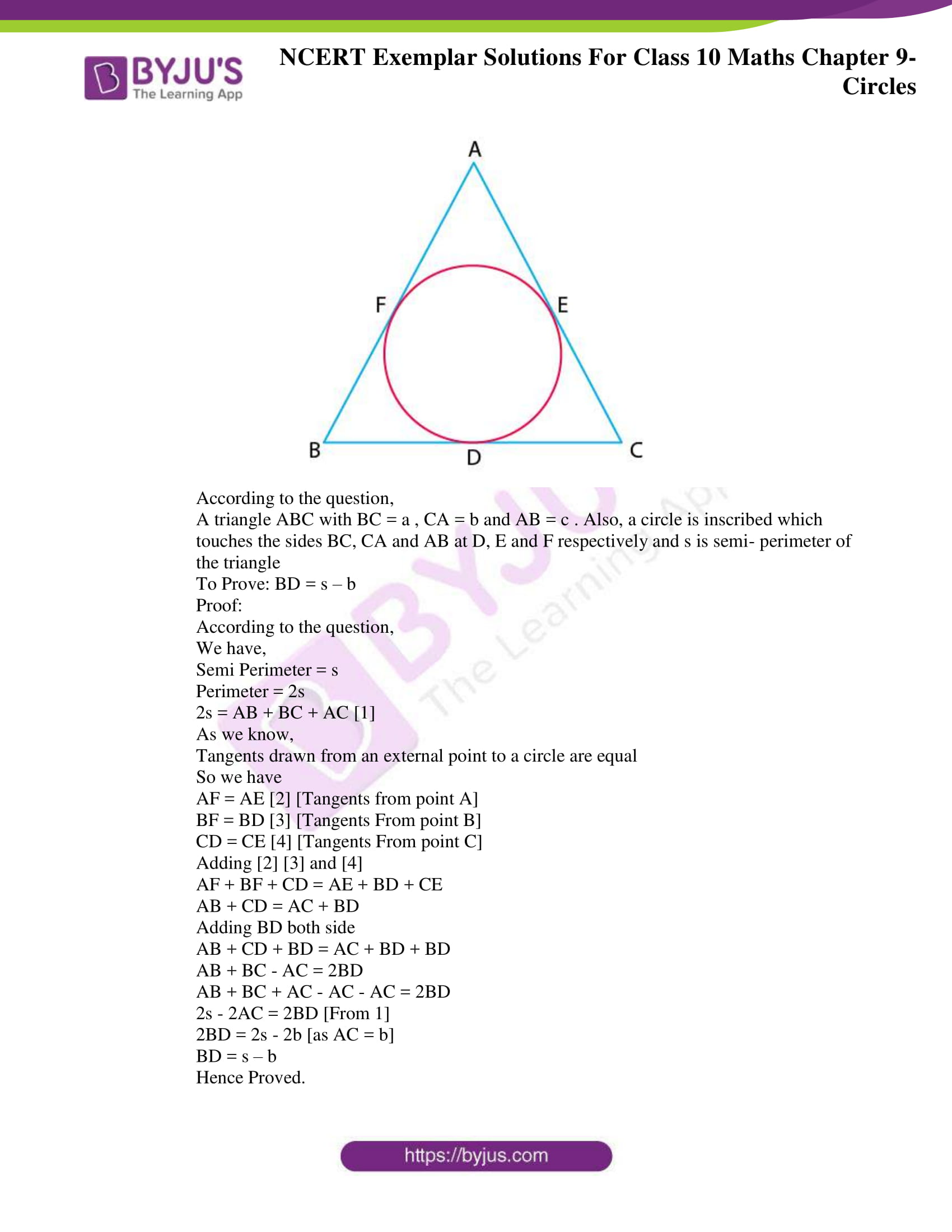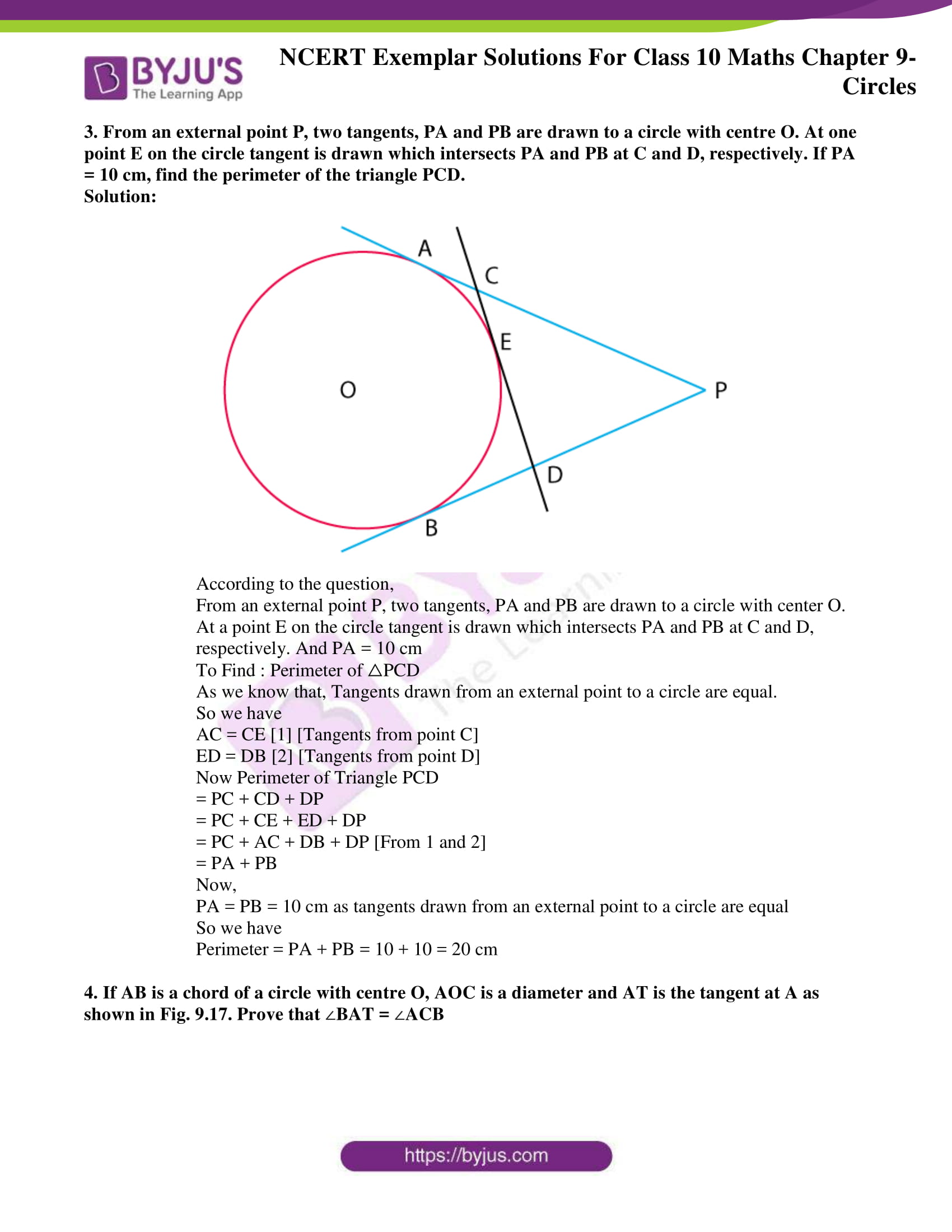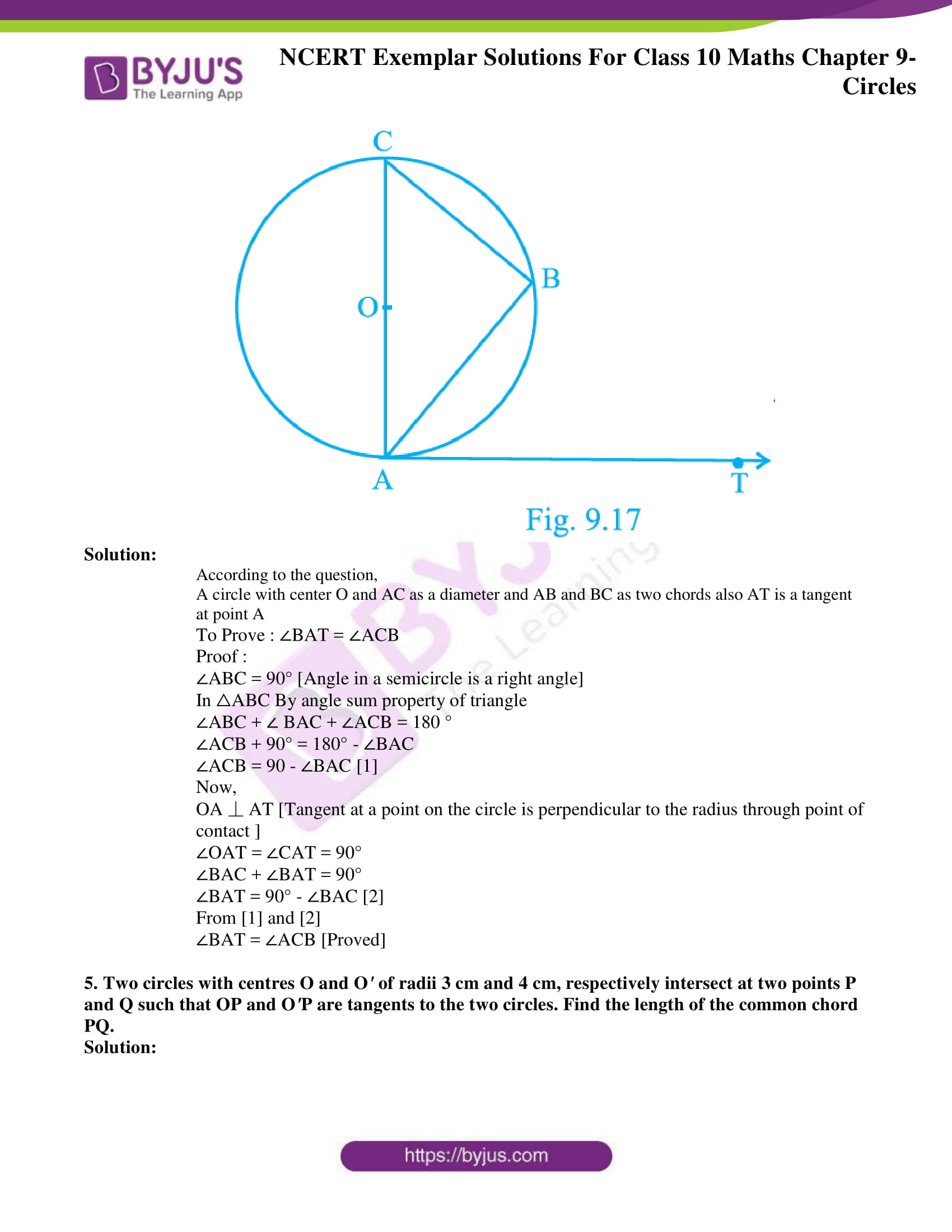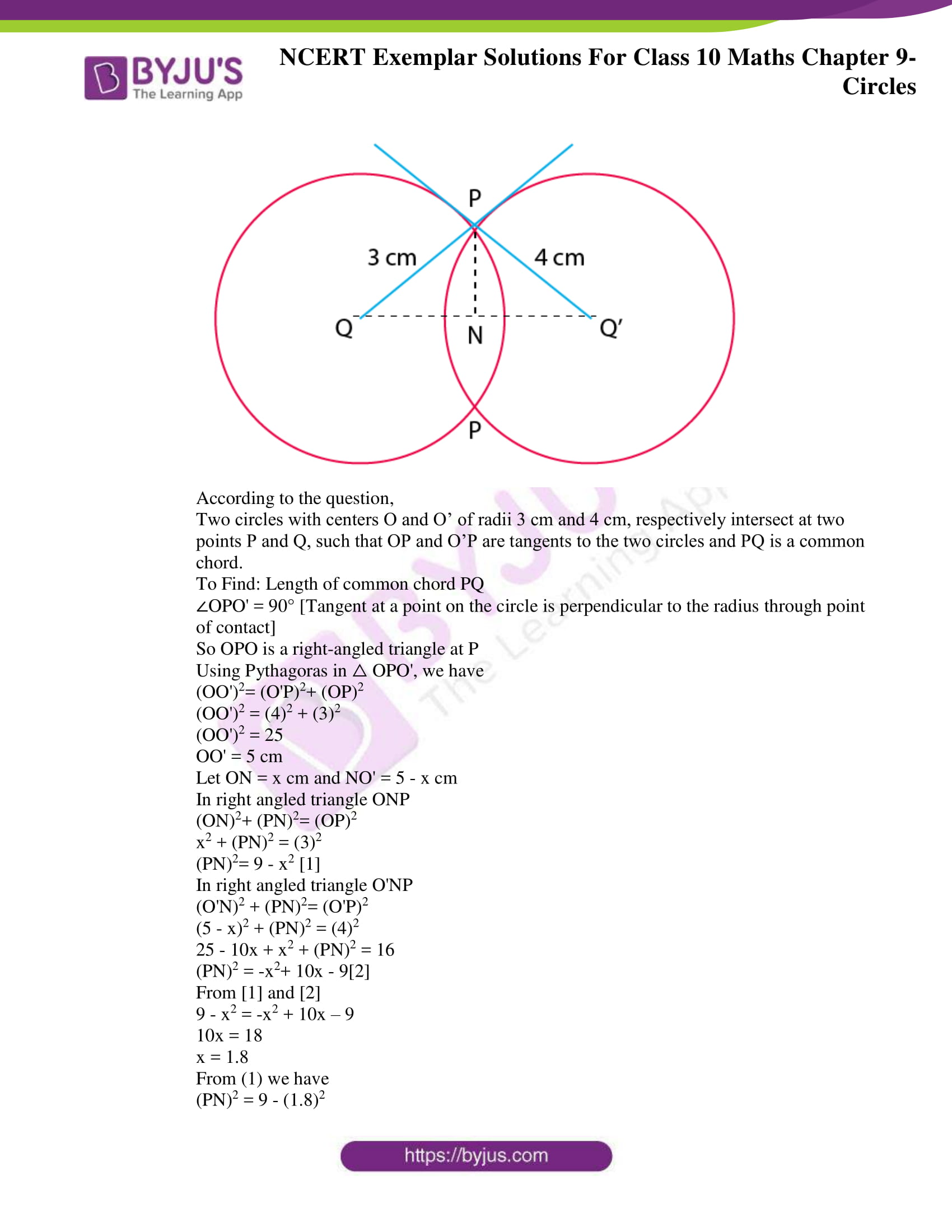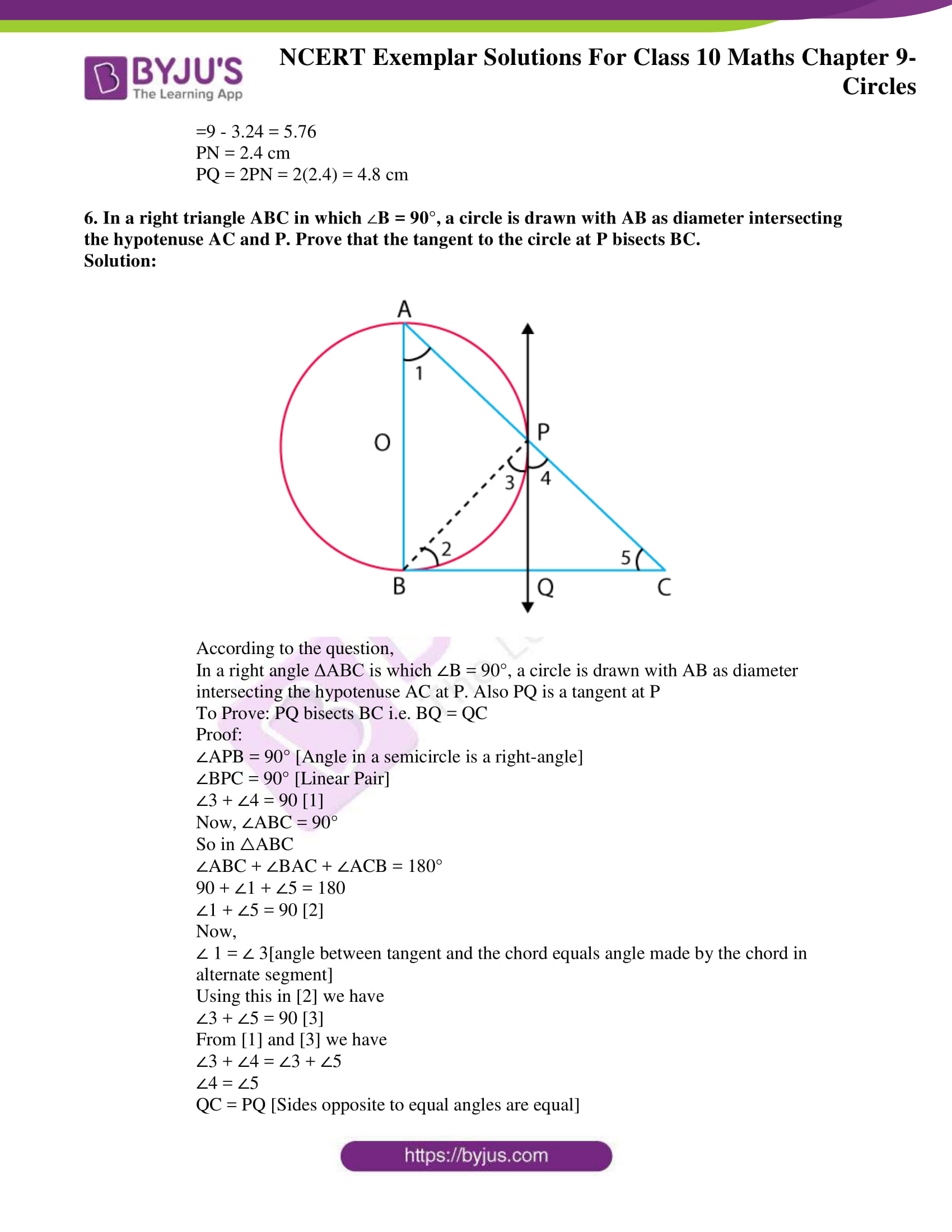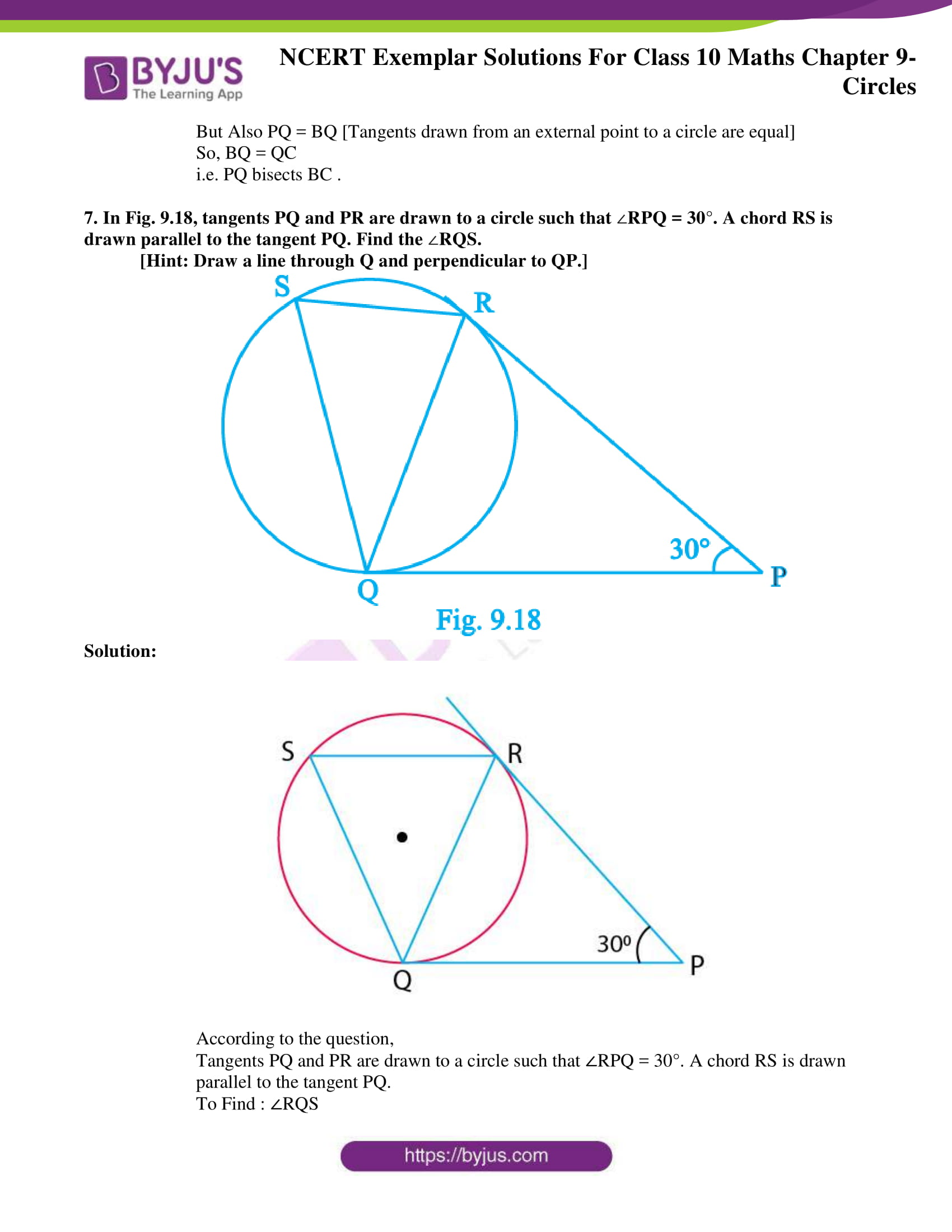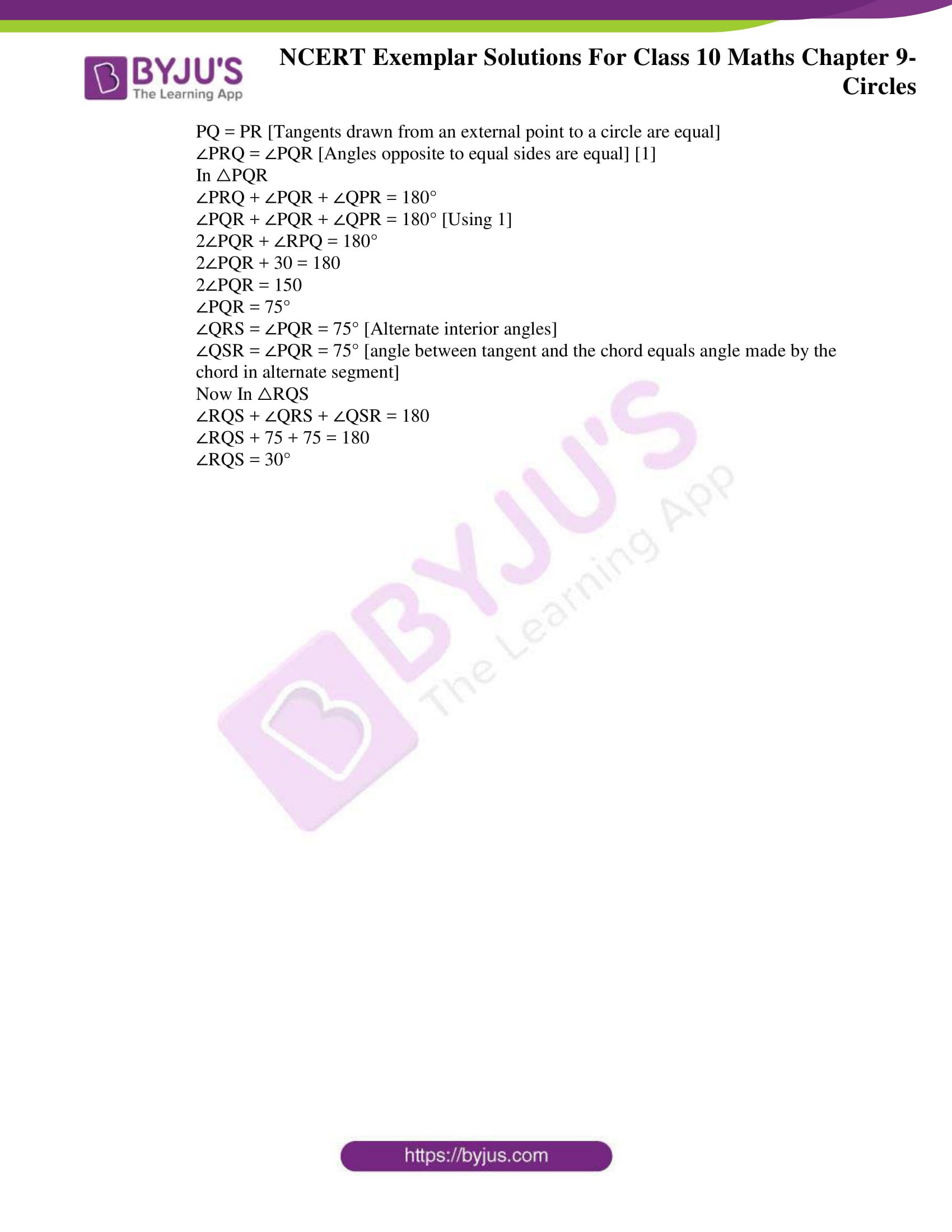## Exercise 9.1 Page No: 102

Choose the correct answer from the given four options in the following questions:

1. If radii of two concentric circles are 4 cm and 5 cm, then the length of each chord of one circle which is tangent to the other circle is

(A) 3 cm (B) 6 cm

(C) 9 cm (D) 1 cm

Solution: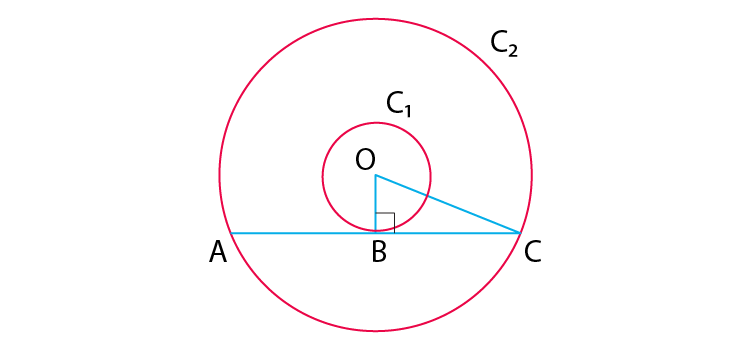According to the question,

OA = 4cm, OB = 5cm

And, OA ⊥ BC

Therefore, OB2 = OA2 + AB2

⇒ 52 = 42 + AB2

⇒ AB = √(25 – 16) = 3cm

⇒ BC = 2AB = 2 × 3cm = 6cm

2. In Fig. 9.3, if AOB = 125°, then COD is equal to

(A) 62.5° (B) 45°

(C) 35° (D) 55°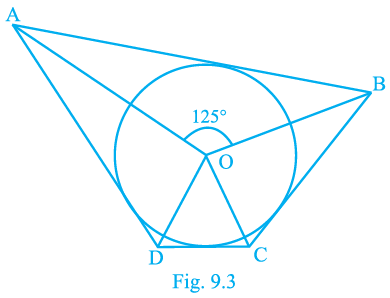Solution:

ABCD is a quadrilateral circumscribing the circle

We know that, the opposite sides of a quadrilateral circumscribing a circle subtend supplementary angles at the center of the circle.

So, we have

∠AOB + ∠COD = 180°

125° + ∠COD = 180°

∠COD = 55°

3. In Fig. 9.4, AB is a chord of the circle and AOC is its diameter such that ACB = 50°. If AT is the tangent to the circle at the point A, then BAT is equal to

(A) 65° (B) 60°

(C) 50° (D) 40°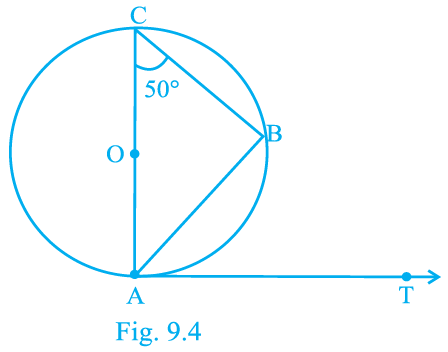Solution:

According to the question,

A circle with centre O, diameter AC and ∠ACB = 50°

AT is a tangent to the circle at point A

Since, angle in a semicircle is a right angle

∠CBA = 90°

By angle sum property of a triangle,

∠ACB + ∠CAB + ∠CBA = 180°

50° + ∠CAB + 90° = 180°

∠CAB = 40° … (1)

Since tangent to at any point on the circle is perpendicular to the radius through point of contact,

We get,

OA ⏊ AT

∠OAT = 90°

∠OAT + ∠BAT = 90°

∠CAT + ∠BAT = 90°

40° + ∠BAT = 90° [from equation (1)]

∠BAT = 50°

4. From a point P which is at a distance of 13 cm from the centre O of a circle of radius 5 cm, the pair of tangents PQ and PR to the circle are drawn. Then the area of the quadrilateral PQOR is

(A) 60 cm2 (B) 65 cm2

(C) 30 cm2 (D) 32.5 cm2

Solution:

Construction: Draw a circle of radius 5 cm with center O.

Let P be a point at a distance of 13 cm from O.

Draw a pair of tangents, PQ and PR.

OQ = OR = radius = 5cm …equation (1)

And OP = 13 cm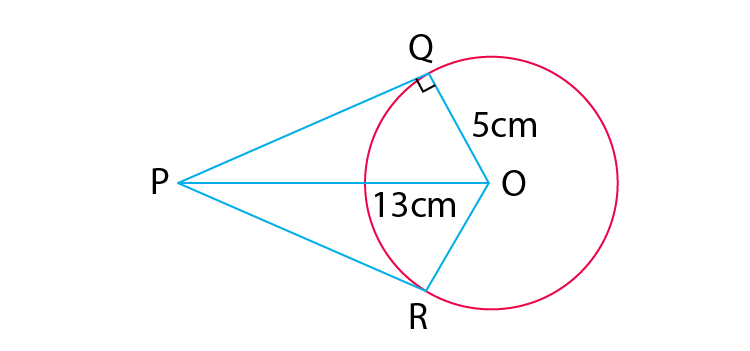We know that, tangent to at any point on the circle is perpendicular to the radius through point of contact,

Hence, we get,

OQ ⏊ PQ and OR ⏊PR

△POQ and △POR are right-angled triangles.

Using Pythagoras Theorem in △PQO,

(Base)2+ (Perpendicular)2= (Hypotenuse)2

(PQ)2 + (OQ)2= (OP)2

(PQ)2 + (5)2 = (13)2

(PQ)2 + 25 = 169

(PQ)2 = 144

PQ = 12 cm

Tangents through an external point to a circle are equal.

So,

PQ = PR = 12 cm … (2)

Therefore, Area of quadrilateral PQRS, A = area of △POQ + area of △POR

Area of right angled triangle = ½ x base x perpendicular

A = (½ x OQ x PQ) + (½ x OR x PR)

A = (½ x 5 x 12) + (½ x 5 x 12)

A = 30 + 30 = 60 cm2

5. At one end A of a diameter AB of a circle of radius 5 cm, tangent XAY is drawn to the circle. The length of the chord CD parallel to XY and at a distance 8 cm from A is

(A) 4 cm (B) 5 cm

(C) 6 cm (D) 8 cm

Solution: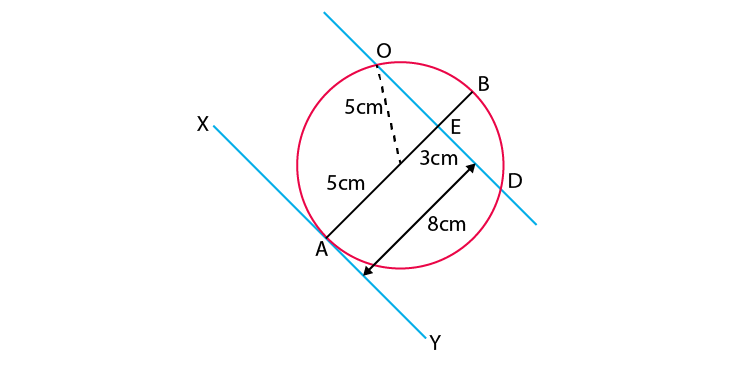According to the question,

Radius of circle, AO=OC = 5cm

AM=8CM

AM=OM+AO

OM =AM-AO

Substituting these values in the equation,

OM= (8-5) =3CM

OM is perpendicular to the chord CD.

In ∆OCM <OMC=90°

By Pythagoras theorem,

OC²=OM²+MC²

Therefore,

CD= 2 ×CM = 8 cm

## Exercise 9.2 Page No: 105

Write ‘True’ or ‘False’ and justify your answer in each of the following:

1. If a chord AB subtends an angle of 60° at the centre of a circle, then angle between the tangents at A and B is also 60°.

Solution:

False

Justification:

For example,

Consider the given figure. In which we have a circle with centre O and AB a chord with ∠AOB = 60°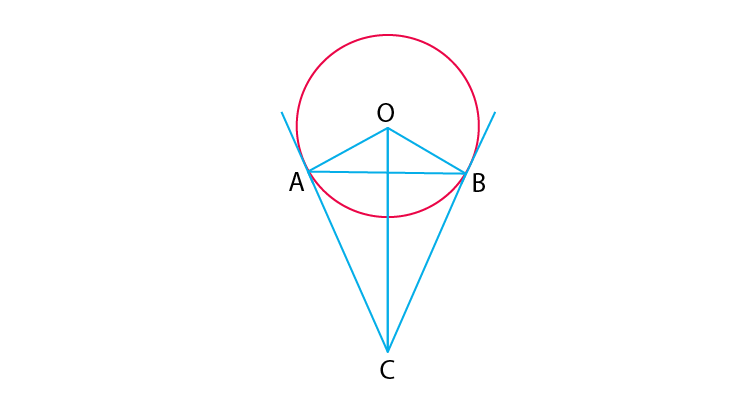Since, tangent to any point on the circle is perpendicular to the radius through point of contact,

We get,

OA ⏊ AC and OB ⏊ CB

∠OBC = ∠OAC = 90° … eq(1)

We get,

∠OBC + ∠OAC + ∠AOB + ∠ACB = 360°

90° + 90° + 60° + ∠ACB = 360°

∠ACB = 120°

Hence, the angle between two tangents is 120°.

Therefore, we can conclude that,

the given statement is false.

2. The length of tangent from an external point on a circle is always greater than the radius of the circle.

Solution:

False

Justification:

Length of tangent from an external point P on a circle may or may not be greater than the radius of the circle.

3. The length of tangent from an external point P on a circle with centre O is always less than OP.

Solution:

True

Justification:

Consider the figure of a circle with centre O.

Let PT be a tangent drawn from external point P.

Now, Joint OT.

OT ⏊ PT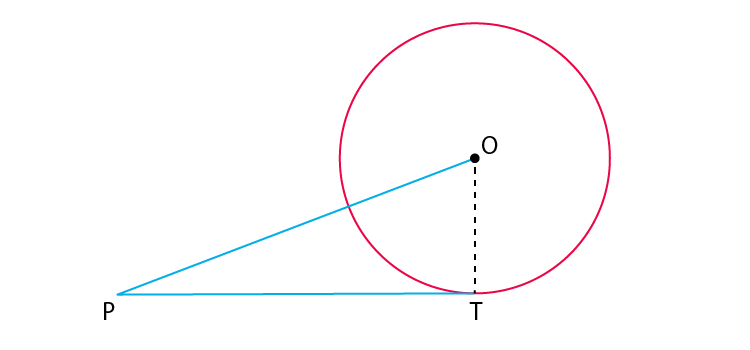We know that,

Tangent at any point on the circle is perpendicular to the radius through point of contact

Hence, OPT is a right-angled triangle formed.

We also know that,

In a right angled triangle, hypotenuse is always greater than any of the two sides of the triangle.

Hence,

OP > PT or PT < OP

Hence, length of tangent from an external point P on a circle with center O is always less than OP.

4. The angle between two tangents to a circle may be 0°.

Solution:

True

Justification:

The angle between two tangents to a circle may be 0°only when both tangent lines coincide or are parallel to each other.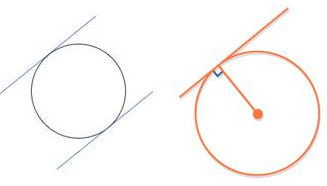5. If angle between two tangents drawn from a point P to a circle of radius a and centre O is 90°, then OP = a2.

Solution:

True.

Tangent is always perpendicular to the radius at the point of contact.

Hence, ∠RPT = 90

If 2 tangents are drawn from an external point, then they are equally inclined to the line segment joining the centre to that point.

Consider the following figure,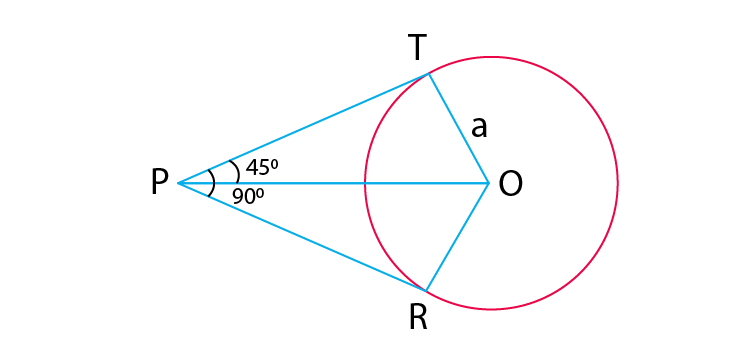From point P, two tangents are drawn.

It is given that, OT = a

And line OP bisects ∠RPT.

So,

∠TPO = ∠RPO = 45o

We know that, OT ⊥ PT

In right-angled triangle OTP,

Sin 45o = OT/OP

= 1/√2 = a/OP

Hence, OP = a√2

## Exercise 9.3 Page No: 107

1. Out of the two concentric circles, the radius of the outer circle is 5 cm and the chord AC of length 8 cm is a tangent to the inner circle. Find the radius of the inner circle.

Solution: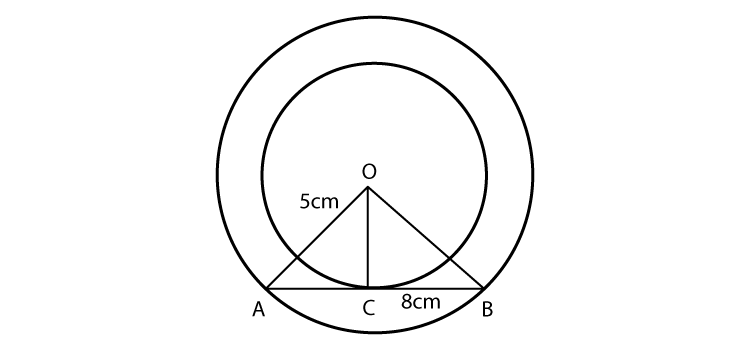From the figure,

Chord AB = 8 cm

OC is perpendicular to the chord AB

AC = CB = 4 cm

In right triangle OCA

OC2 + CA2 = OA2

OC2 = 5– 42 = 25 – 16 = 9

OC = 3 cm

2. Two tangents PQ and PR are drawn from an external point to a circle with centre O. Prove that QORP is a cyclic quadrilateral.

Solution: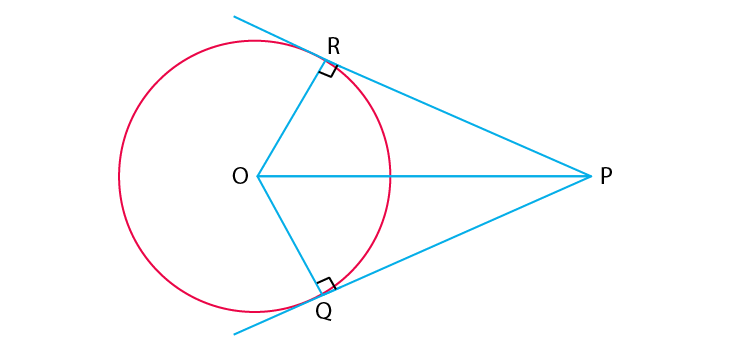We know that,

Radius ⊥ Tangent = OR ⊥ PR

i.e., ∠ORP = 90°

Likewise,

Radius ⊥ Tangent = OQ ⊥PQ

∠OQP = 90°

Sum of all interior angles = 360º

∠ORP + ∠RPQ+ ∠PQO + ∠QOR = 360º

90º + ∠RPQ + 90º + ∠QOR = 360º

Hence, ∠O + ∠P = 180o

3. If from an external point B of a circle with centre O, two tangents BC and BD are drawn such that angle DBC = 120°, prove that BC + BD = BO, i.e., BO = 2BC.

Solution:

According to the question,

By RHS rule,

ΔOBC and ΔOBD are congruent

By CPCT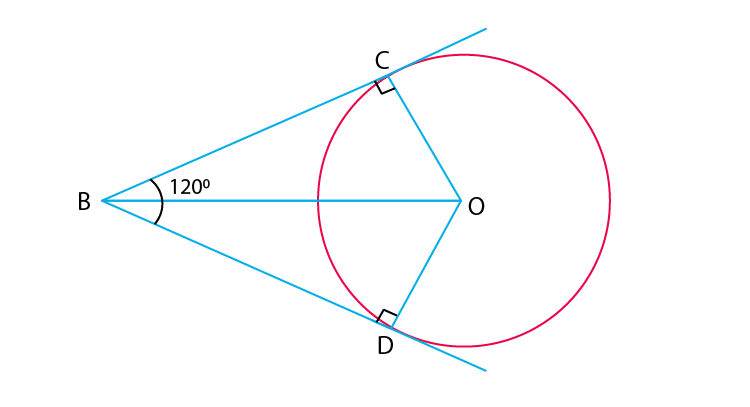∠OBC and ∠ OBD are equal

Therefore,

∠OBC = ∠OBD =60°

In triangle OBC,

cos 60°=BC/OB

½ =BC/OB

OB=2BC

Hence proved

4. Prove that the centre of a circle touching two intersecting lines lies on the angle bisector of the lines.

Solution: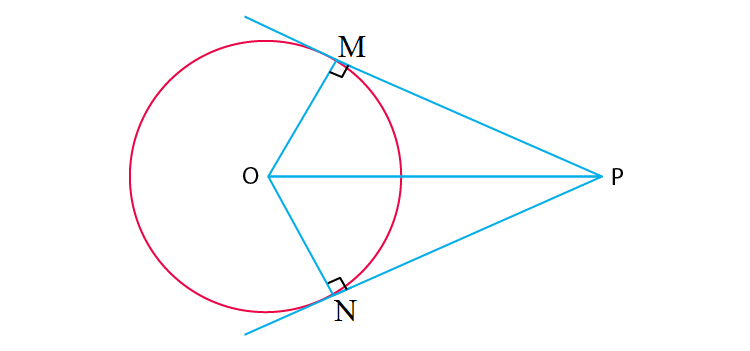Let the lines be l1 and l2.

Assume that O touches l₁ and l₂ at M and N,

We get,

OM = ON (Radius of the circle)

Therefore,

From the centre ”O” of the circle, it has equal distance from l₁ & l₂.

In Δ OPM & OPN,

OM = ON (Radius of the circle)

∠OMP = ∠ONP (As, Radius is perpendicular to its tangent)

OP = OP (Common sides)

Therefore,

Δ OPM = ΔOPN (SSS congruence rule)

By C.P.C.T,

∠MPO = ∠NPO

So, l bisects ∠MPN.

Therefore, O lies on the bisector of the angle between l₁ & l₂ .

Hence, we prove that the centre of a circle touching two intersecting lines lies on the angle bisector of the lines.

5. In Fig. 9.13, AB and CD are common tangents to two circles of unequal radii. Prove that AB = CD.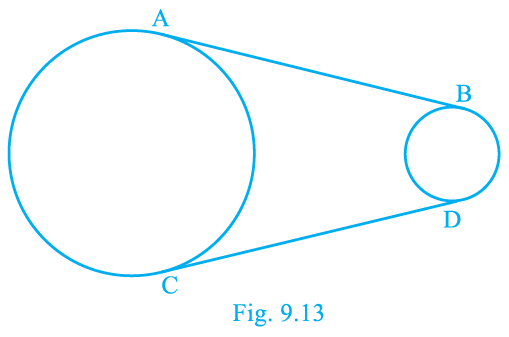Solution:

According to the question,

AB = CD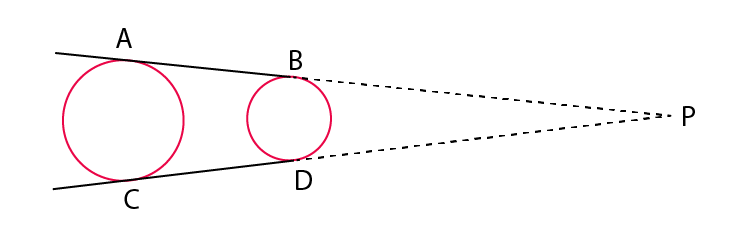Construction: Produce AB and CD, to intersect at P.

Proof:

Consider the circle with greater radius.

Tangents drawn from an external point to a circle are equal

AP = CP …(1)

Also,

Consider the circle with smaller radius.

Tangents drawn from an external point to a circle are equal

BP = BD …(2)

Subtract Equation (2) from (1). We Get

AP – BP = CP – BD

AB = CD

Hence Proved.

Exercise 9.4 Page No: 110

1. If a hexagon ABCDEF circumscribe a circle, prove that AB + CD + EF = BC + DE + FA.

Solution: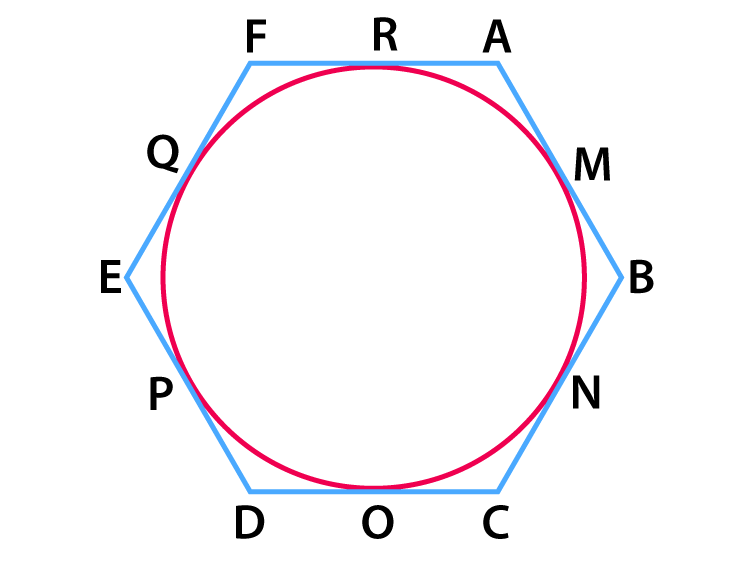According to the question,

A Hexagon ABCDEF circumscribe a circle.

To prove:

AB + CD + EF = BC + DE + FA

Proof:

Tangents drawn from an external point to a circle are equal.

Hence, we have

AM = RA … eq 1 [tangents from point A]

BM = BN … eq 2 [tangents from point B]

CO = NC … eq 3 [tangents from point C]

OD = DP … eq 4 [tangents from point D]

EQ = PE … eq 5 [tangents from point E]

QF = FR … eq 6 [tangents from point F] [eq 1]+[eq 2]+[eq 3]+[eq 4]+[eq 5]+[eq 6]

AM + BM + CO + OD + EQ + QF = RA + BN + NC + DP + PE + FR

On rearranging, we get,

(AM + BM) + (CO + OD) + (EQ + QF) = (BN + NC) + (DP + PE) + (FR + RA)

AB + CD + EF = BC + DE + FA

Hence Proved!

2. Let s denote the semi-perimeter of a triangle ABC in which BC = a, CA = b, AB = c. If a circle touches the sides BC, CA, AB at D, E, F, respectively, prove that BD = s b.

Solution: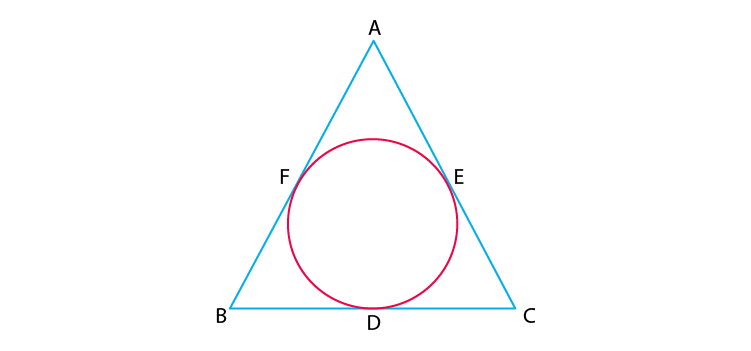According to the question,

A triangle ABC with BC = a , CA = b and AB = c . Also, a circle is inscribed which touches the sides BC, CA and AB at D, E and F respectively and s is semi- perimeter of the triangle

To Prove: BD = s – b

Proof:

According to the question,

We have,

Semi Perimeter = s

Perimeter = 2s

2s = AB + BC + AC 

As we know,

Tangents drawn from an external point to a circle are equal

So we have

AF = AE  [Tangents from point A]

BF = BD  [Tangents From point B]

CD = CE  [Tangents From point C]

AF + BF + CD = AE + BD + CE

AB + CD = AC + BD

AB + CD + BD = AC + BD + BD

AB + BC – AC = 2BD

AB + BC + AC – AC – AC = 2BD

2s – 2AC = 2BD [From 1]

2BD = 2s – 2b [as AC = b]

BD = s – b

Hence Proved.

3. From an external point P, two tangents, PA and PB are drawn to a circle with centre O. At one point E on the circle tangent is drawn which intersects PA and PB at C and D, respectively. If PA = 10 cm, find the perimeter of the triangle PCD.

Solution: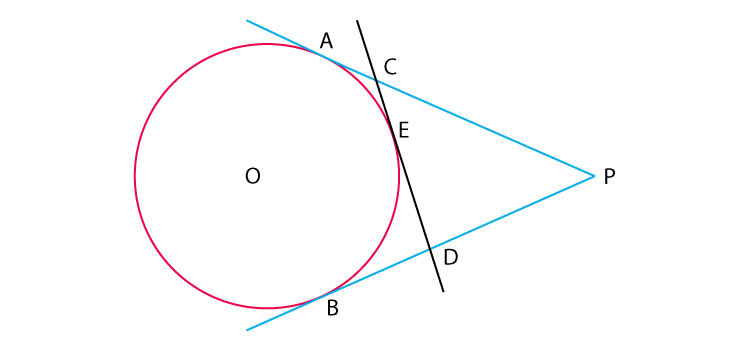According to the question,

From an external point P, two tangents, PA and PB are drawn to a circle with center O. At a point E on the circle tangent is drawn which intersects PA and PB at C and D, respectively. And PA = 10 cm

To Find : Perimeter of △PCD

As we know that, Tangents drawn from an external point to a circle are equal.

So we have

AC = CE  [Tangents from point C]

ED = DB  [Tangents from point D]

Now Perimeter of Triangle PCD

= PC + CD + DP

= PC + CE + ED + DP

= PC + AC + DB + DP [From 1 and 2]

= PA + PB

Now,

PA = PB = 10 cm as tangents drawn from an external point to a circle are equal

So we have

Perimeter = PA + PB = 10 + 10 = 20 cm

4. If AB is a chord of a circle with centre O, AOC is a diameter and AT is the tangent at A as shown in Fig. 9.17. Prove that BAT = ACB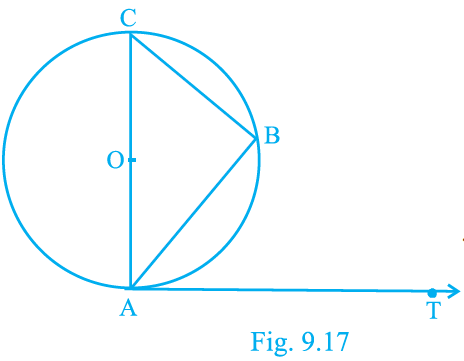Solution:

According to the question,

A circle with center O and AC as a diameter and AB and BC as two chords also AT is a tangent at point A

To Prove : ∠BAT = ∠ACB

Proof :

∠ABC = 90° [Angle in a semicircle is a right angle]

In △ABC By angle sum property of triangle

∠ABC + ∠ BAC + ∠ACB = 180 °

∠ACB + 90° = 180° – ∠BAC

∠ACB = 90 – ∠BAC 

Now,

OA ⏊ AT [Tangent at a point on the circle is perpendicular to the radius through point of contact ]

∠OAT = ∠CAT = 90°

∠BAC + ∠BAT = 90°

∠BAT = 90° – ∠BAC 

From  and 

∠BAT = ∠ACB [Proved]

5. Two circles with centres O and Oof radii 3 cm and 4 cm, respectively intersect at two points P and Q such that OP and OP are tangents to the two circles. Find the length of the common chord PQ.

Solution: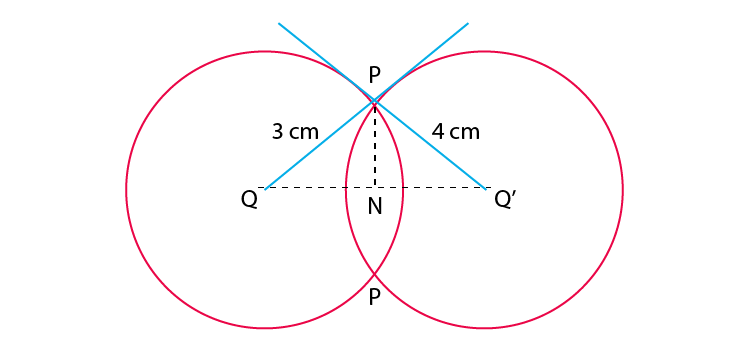According to the question,

Two circles with centers O and O’ of radii 3 cm and 4 cm, respectively intersect at two points P and Q, such that OP and O’P are tangents to the two circles and PQ is a common chord.

To Find: Length of common chord PQ

∠OPO’ = 90° [Tangent at a point on the circle is perpendicular to the radius through point of contact]

So OPO is a right-angled triangle at P

Using Pythagoras in △ OPO’, we have

(OO’)2= (O’P)2+ (OP)2

(OO’)2 = (4)2 + (3)2

(OO’)2 = 25

OO’ = 5 cm

Let ON = x cm and NO’ = 5 – x cm

In right angled triangle ONP

(ON)2+ (PN)2= (OP)2

x2 + (PN)2 = (3)2

(PN)2= 9 – x2 

In right angled triangle O’NP

(O’N)2 + (PN)2= (O’P)2

(5 – x)2 + (PN)2 = (4)2

25 – 10x + x2 + (PN)2 = 16

(PN)2 = -x2+ 10x – 9

From  and 

9 – x2 = -x2 + 10x – 9

10x = 18

x = 1.8

From (1) we have

(PN)2 = 9 – (1.8)2

=9 – 3.24 = 5.76

PN = 2.4 cm

PQ = 2PN = 2(2.4) = 4.8 cm

6. In a right triangle ABC in which B = 90°, a circle is drawn with AB as diameter intersecting the hypotenuse AC and P. Prove that the tangent to the circle at P bisects BC.

Solution: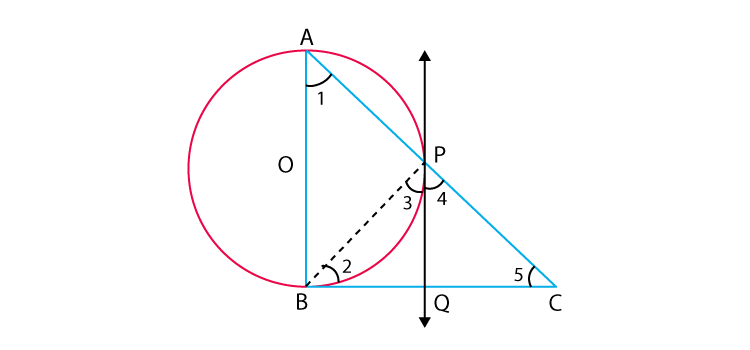According to the question,

In a right angle ΔABC is which ∠B = 90°, a circle is drawn with AB as diameter intersecting the hypotenuse AC at P. Also PQ is a tangent at P

To Prove: PQ bisects BC i.e. BQ = QC

Proof:

∠APB = 90° [Angle in a semicircle is a right-angle]

∠BPC = 90° [Linear Pair]

∠3 + ∠4 = 90 

Now, ∠ABC = 90°

So in △ABC

∠ABC + ∠BAC + ∠ACB = 180°

90 + ∠1 + ∠5 = 180

∠1 + ∠5 = 90 

Now,

∠ 1 = ∠ 3[angle between tangent and the chord equals angle made by the chord in alternate segment]

Using this in  we have

∠3 + ∠5 = 90 

From  and  we have

∠3 + ∠4 = ∠3 + ∠5

∠4 = ∠5

QC = PQ [Sides opposite to equal angles are equal]

But Also PQ = BQ [Tangents drawn from an external point to a circle are equal]

So, BQ = QC

i.e. PQ bisects BC .

7. In Fig. 9.18, tangents PQ and PR are drawn to a circle such that RPQ = 30°. A chord RS is drawn parallel to the tangent PQ. Find the RQS.

[Hint: Draw a line through Q and perpendicular to QP.]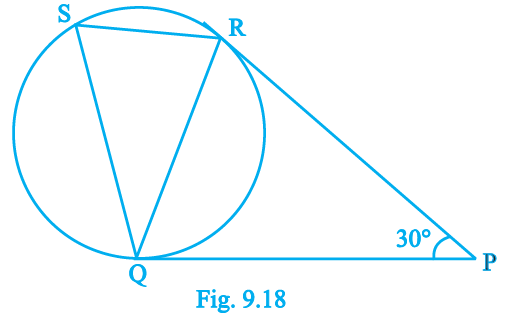Solution: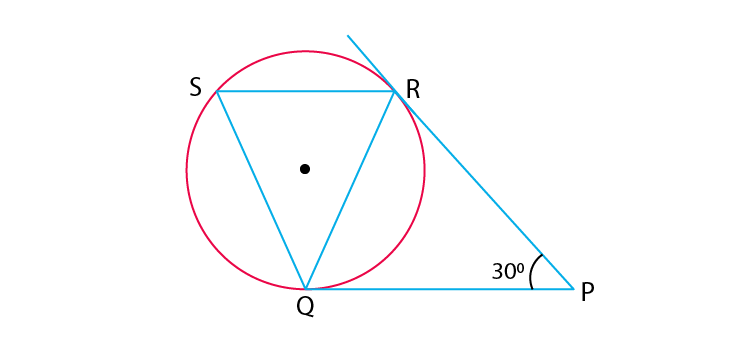According to the question,

Tangents PQ and PR are drawn to a circle such that ∠RPQ = 30°. A chord RS is drawn parallel to the tangent PQ.

To Find : ∠RQS

PQ = PR [Tangents drawn from an external point to a circle are equal]

∠PRQ = ∠PQR [Angles opposite to equal sides are equal] 

In △PQR

∠PRQ + ∠PQR + ∠QPR = 180°

∠PQR + ∠PQR + ∠QPR = 180° [Using 1]

2∠PQR + ∠RPQ = 180°

2∠PQR + 30 = 180

2∠PQR = 150

∠PQR = 75°

∠QRS = ∠PQR = 75° [Alternate interior angles]

∠QSR = ∠PQR = 75° [angle between tangent and the chord equals angle made by the chord in alternate segment]

Now In △RQS

∠RQS + ∠QRS + ∠QSR = 180

∠RQS + 75 + 75 = 180

∠RQS = 30°

To get more practice for the exam, students are also provided with online reading materials such as notes, exemplar books, NCERT Maths Solutions for 10th Class and question papers, which they can make the best use of it. And they can solve sample papers and previous year question papers to get an idea of the type of questions asked from chapter Circles.

Download BYJU’S app to get personalized videos, explaining different types of Maths topics such as circles and experience a new method of learning to understand the concepts in an easy way.

## Frequently Asked Questions on NCERT Exemplar Solutions for Class 10 Maths Chapter 9

### What is tangent to a circle in Chapter 9 of NCERT Exemplar Solutions for Class 10 Maths?

A tangent to a circle is a line which intersects the circle at only one point. The common point between the tangent and the circle is called the point of contact. This is one of the important chapters in Class 10 as these concepts have numerous applications in our daily life. Students are advised to solve this chapter using the solutions designed by the experts at BYJU’S to score well in the Class 10 exams.

### What are the concepts covered in Chapter 9 of NCERT Exemplar Solutions for Class 10 Maths?

This chapter covers the following topics of circles given below:
1. Tangent to a given circle
2. Number of tangents drawn from a point on a circle
3. Proof of -Tangents at the end of the diameter of a circle are parallel
Students can understand these concepts more efficiently by using the solutions framed by the expert faculty at BYJU’S.

### Does NCERT Exemplar Solutions for Class 10 Maths Chapter 9 have answers for all the textbook questions?

The NCERT Exemplar Solutions for Class 10 Maths Chapter 9 is available in PDF format designed by the subject experts. These solutions are completely based on the syllabus created by the CBSE board and covers all the important concepts for the exam. The textbook problems are solved in a stepwise manner as per the marks weightage in the board exams. Both chapter wise and exercise wise PDF links are present in BYJU’S which can be accessed by the students to get their doubts clarified instantly.